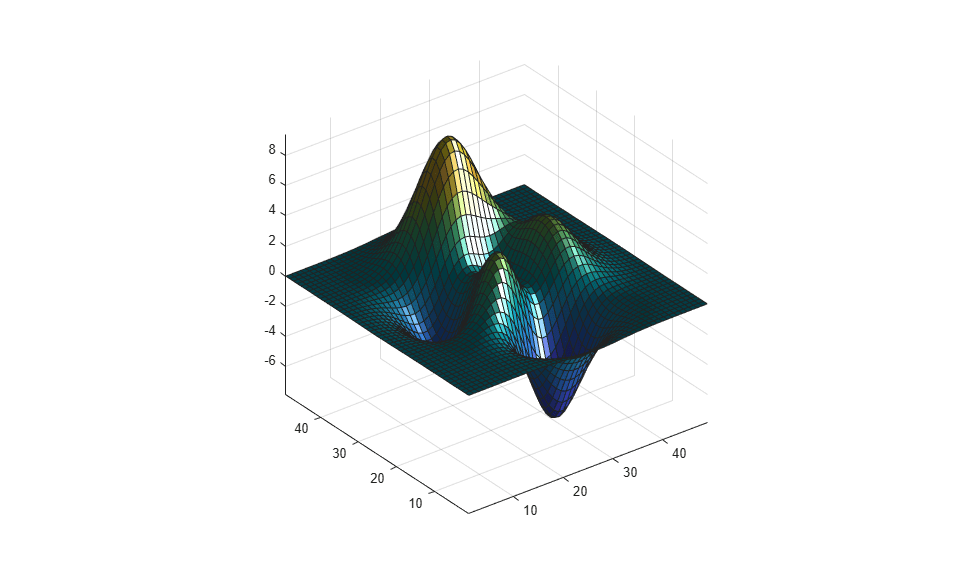# lightangle

Create or position light object in spherical coordinates

## Syntax

```lightangle(az,el) lightangle(ax,az,el) lgt = lightangle(...) lightangle(lgt,az,el) [az,el] = lightangle(lgt) ```

## Description

`lightangle(az,el)` creates a light at the position specified by azimuth and elevation. `az` is the azimuthal (horizontal) rotation and `el` is the vertical elevation (both in degrees). The interpretation of azimuth and elevation is the same as that of the `view` command.

`lightangle(ax,az,el)` creates a light in the axes specified by `ax` instead of the current axes.

`lgt = lightangle(...)` creates a light and returns the light object as `lgt`. You can specify an output argument with any of the previous input argument combinations.

`lightangle(lgt,az,el)` sets the position of the light specified by `lgt`.

`[az,el] = lightangle(lgt)` returns the azimuth and elevation of the light specified by `lgt`.

## Examples

collapse all

Create a surface. Add a light and move the position of the light.

```surf(peaks) axis vis3d h = light; for az = -50:10:50 lightangle(h,az,30) pause(.1) end ```## Tips

By default, when a light is created, its style is `infinite`. If the light handle passed in to `lightangle` refers to a local light, the distance between the light and the camera target is preserved as the position is changed.

## Version History

Introduced before R2006a